NEET  >  P. Bahadur Test: Chemical Kinetics

# P. Bahadur Test: Chemical Kinetics

Test Description

## 30 Questions MCQ Test Chemistry Class 12 | P. Bahadur Test: Chemical Kinetics

P. Bahadur Test: Chemical Kinetics for NEET 2023 is part of Chemistry Class 12 preparation. The P. Bahadur Test: Chemical Kinetics questions and answers have been prepared according to the NEET exam syllabus.The P. Bahadur Test: Chemical Kinetics MCQs are made for NEET 2023 Exam. Find important definitions, questions, notes, meanings, examples, exercises, MCQs and online tests for P. Bahadur Test: Chemical Kinetics below.
Solutions of P. Bahadur Test: Chemical Kinetics questions in English are available as part of our Chemistry Class 12 for NEET & P. Bahadur Test: Chemical Kinetics solutions in Hindi for Chemistry Class 12 course. Download more important topics, notes, lectures and mock test series for NEET Exam by signing up for free. Attempt P. Bahadur Test: Chemical Kinetics | 30 questions in 45 minutes | Mock test for NEET preparation | Free important questions MCQ to study Chemistry Class 12 for NEET Exam | Download free PDF with solutions
 1 Crore+ students have signed up on EduRev. Have you?
P. Bahadur Test: Chemical Kinetics - Question 1

### The time for half life period of a certain reaction A → Products is 1 h. When the initial concentration of the reactant 'A' is 2.0 mol L–1, how much time does it take for its concentration to come from 0.50 to 0.25 mol L–1, if it is a zero order reaction?

Detailed Solution for P. Bahadur Test: Chemical Kinetics - Question 1

The correct answer is Option A.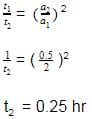P. Bahadur Test: Chemical Kinetics - Question 2

### N205 → 2NO2 + O2 When N205 decompose, its t12 does not change with its changing pressure during the reaction, so which one is the correct representation for "pressure of 2NO2" vs lime° during the reaction when initial N205 is equals to Po

Detailed Solution for P. Bahadur Test: Chemical Kinetics - Question 2

The correct sentence is option C
Since the half-life period is independent of the changing pressure, the reaction is of the first order.
The integrated rate law is (PN2​O5​​)t​=(P​oN2​O5​)0e−kt
Thus the partial pressure of N2​O5​ decreases exponentially with time and the partial pressure of NO2​ increases exponentially with time.
Hence, the correct graph is represented by option C.

P. Bahadur Test: Chemical Kinetics - Question 3

### In a hypothetical reaction A(aq)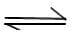2B(aq) + C(aq)    (Ist order decomposition) 'A' is optically active (dextro-rototory) while 'B' and 'C' are optically inactive but 'B' takes part in a titration reaction (fast reaction) with H202. Hence the progress of reaction can be monitored by measuring rotation of plane of plane polarised light or by measuring volume of H202 consumed in titration.  In an experiment the optical rotation was found to be 0 = 30° at t = 20 min and 8 =15° at t= 50 min. from start of the reaction. If the progress would have been monitored by titration method, volume of H202 consumed at t = 30 min. (from start) is 30 ml then volume of H202 consumed at t = 90 min will be:

Detailed Solution for P. Bahadur Test: Chemical Kinetics - Question 3

(B) As only A is optically active. So conc. of A at t =20min ∝ 30°

While concentration of A at t= 50min ∝ mc 15°

So conc. has deceased to half oils value in 30 min, so t1/2=30 min.

So volume consumed of H202 at t =30 min =t1/2 ,is according to 50% production of B at t= 90 min. production of B=87.5%(Threehalflives)

So volume consumed =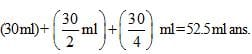P. Bahadur Test: Chemical Kinetics - Question 4

The elementary reaction A + B  products has k = 2 x 10-5 M-1 S-1 at a temperature of 27°C. Several experimental runs are carried out using stoichiometric proportion. The reaction has a temperature coefficient value of 2.0. At what temperature should the reaction be carried out if inspite of halving the concentrations,the rate of reaction is desired to be 50% higher than a previous run.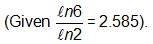Detailed Solution for P. Bahadur Test: Chemical Kinetics - Question 4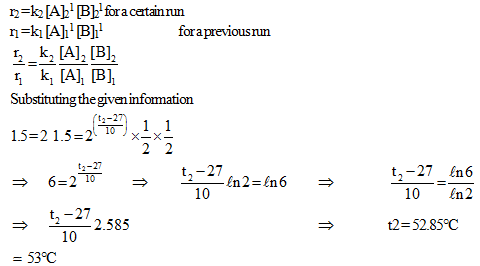P. Bahadur Test: Chemical Kinetics - Question 5

At a certain temperature, the first order rate constant k1 is found to be smaller than the second order rate constant k2. If the energy of activation E1 of the first order reaction is greater than energy of activation E2 of the second order reaction, then with increase in temperature.

Detailed Solution for P. Bahadur Test: Chemical Kinetics - Question 5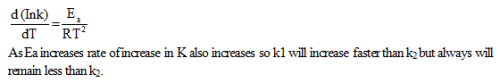P. Bahadur Test: Chemical Kinetics - Question 6

For the system

A2(g) + B2(g)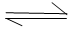2AB(g), AH = – 80 KJ/ mole

If the activation energy for the forward step is 100 kcal /mole. What is the ratio of temperature at which the forward and backward reaction shows the same % change of rate constant per degree rise of temperature ? (1 cal = 4.2 J)

Detailed Solution for P. Bahadur Test: Chemical Kinetics - Question 6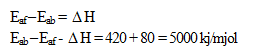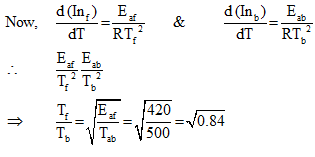P. Bahadur Test: Chemical Kinetics - Question 7

All reactions are of Ist order

At time t = ti (ti > 0)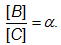Therefore at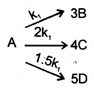time     t = t2 (where t2 > ti)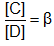which of the following is correct.

Detailed Solution for P. Bahadur Test: Chemical Kinetics - Question 7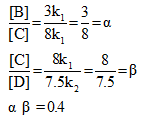P. Bahadur Test: Chemical Kinetics - Question 8

In the formation of HBr from H2 & Br2 , following mechanism is observed.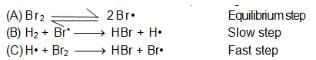Calculate the rate of reaction, it concentration of hydrogen is twice that of bromine and the rate constant is equal to one rutherford. If conc. of bromine is 1 M.

Detailed Solution for P. Bahadur Test: Chemical Kinetics - Question 8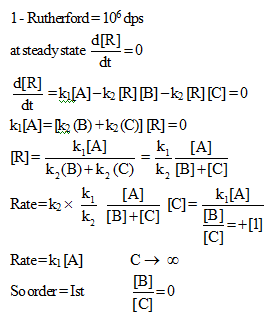P. Bahadur Test: Chemical Kinetics - Question 9

A graph between log t112 and log a (abscissa) a being the initial concentration of A in the reaction For reaction A 4 Product, is the rate law is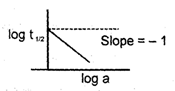Detailed Solution for P. Bahadur Test: Chemical Kinetics - Question 9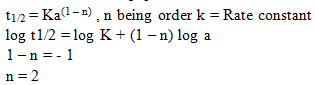P. Bahadur Test: Chemical Kinetics - Question 10

The reaction A (g) + B (g)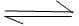C (g) + D (g) is elementry 2nd order reaction. When started with equimolar amounts of A and B, at equilibrium, it is found that the concentration of A is twice that of C. Specific rate for forward reaction is 2 x 10-3 mol-1 Lsec-1. The specific rate constant for backward reaction is :

Detailed Solution for P. Bahadur Test: Chemical Kinetics - Question 10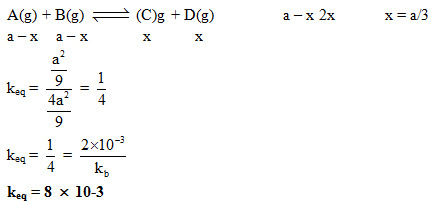P. Bahadur Test: Chemical Kinetics - Question 11

For the reaction R – X + OH- ROH + X- The Rate is given of Rate = 5.0x 10-5[R – X [OH-] + 0.20x 10-5[R – X] what percentage R – X Reaction by SN2 mechanism when [OH-–] = 1.0x 10-2 M

Detailed Solution for P. Bahadur Test: Chemical Kinetics - Question 11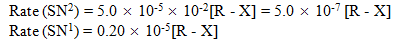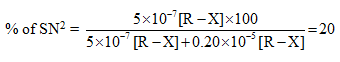P. Bahadur Test: Chemical Kinetics - Question 12

For a 1st order reaction (gaseous) (cont. V, T)

a A  → 4 (b 1) B + 1 C

(with b > a) the pressure of the system rose  by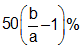in a time of 10 min. The half life of the reaction is therefore.

Detailed Solution for P. Bahadur Test: Chemical Kinetics - Question 12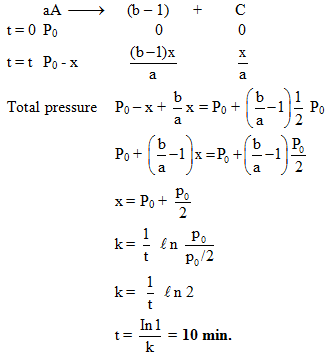P. Bahadur Test: Chemical Kinetics - Question 13

For a certain reaction the variation of the rate constant with temperature is given by the equation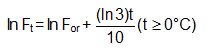The value of tile temperature coefficient of the reaction is

Detailed Solution for P. Bahadur Test: Chemical Kinetics - Question 13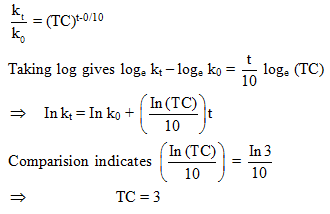P. Bahadur Test: Chemical Kinetics - Question 14

For a reaction A + B → C + D if the concentration of A is doubled without altering the concentration of B, the rate gets doubled. If the concentration of B is increased by nine times without altering the concentration of A, the rate gets tripled. The order of the reaction is

Detailed Solution for P. Bahadur Test: Chemical Kinetics - Question 14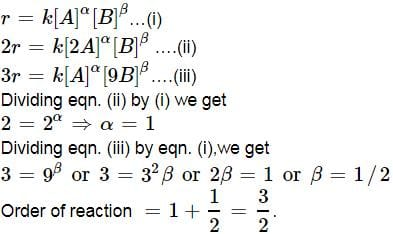P. Bahadur Test: Chemical Kinetics - Question 15

A reaction takes place in three steps ; the rate constants are Br k2 and k3.The overall rate constant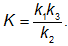If energies of activation are 40 , 30 and 20 kJ, the overall energy of activation is (assumming 'A' to be constant for all )

Detailed Solution for P. Bahadur Test: Chemical Kinetics - Question 15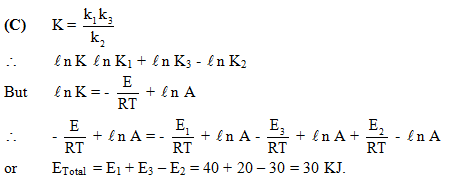P. Bahadur Test: Chemical Kinetics - Question 16

The inversion of cane sugar proceeds with half life of 600 minute at pH = 5 for any concentration of sugar, However at pH = 6, the half life changes to 60 minute, The rate law expression for sugar inversion can be written as

Detailed Solution for P. Bahadur Test: Chemical Kinetics - Question 16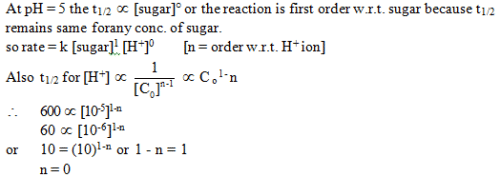P. Bahadur Test: Chemical Kinetics - Question 17

Some graph are sketch for the reaction AB (assuming different orders).Where 'α' represent the degree of dissociation.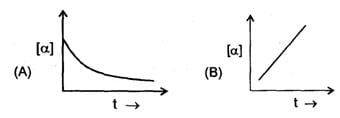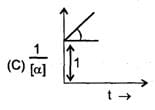The order of reaction is respectively.

Detailed Solution for P. Bahadur Test: Chemical Kinetics - Question 17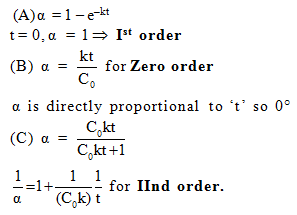P. Bahadur Test: Chemical Kinetics - Question 18

Compounds A and B react with a common reagent with first order kinetics in both cases. If 99% of A must react before 1% of B has reacted. What is the minimum ratio for their respective rate constants?

Detailed Solution for P. Bahadur Test: Chemical Kinetics - Question 18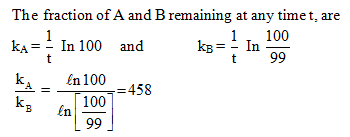P. Bahadur Test: Chemical Kinetics - Question 19

The activity per ml of a solution of radioactive substance is x. How much water be added to 200 ml of this solution so that the acitivity falls to x/20 per ml after 4 half-lives ?

Detailed Solution for P. Bahadur Test: Chemical Kinetics - Question 19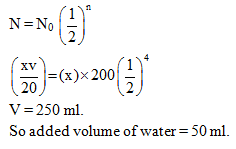P. Bahadur Test: Chemical Kinetics - Question 20

A hypothetical reaction :

A2 + B2 → 2AB Follows mechanism as given below :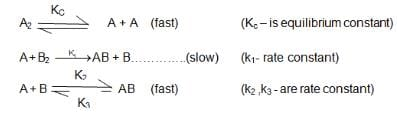The order of overall reaction is

Detailed Solution for P. Bahadur Test: Chemical Kinetics - Question 20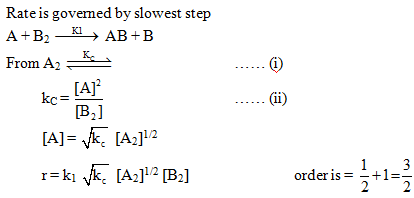P. Bahadur Test: Chemical Kinetics - Question 21

For the decomposition of H202(aq) it was found that Vo2 (t =15 min.) was 100 mL (at 0°C and 1 atm) while Vo2(maximum) was 200 mL (at 0°C and 2 atm). If the same reaction had been followed by the titration method and if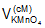(t = 0) had been 40 mL, what would(t = 15 min) have been ?

Detailed Solution for P. Bahadur Test: Chemical Kinetics - Question 21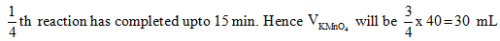P. Bahadur Test: Chemical Kinetics - Question 22

The high temperature (z, 1200K) decomposition of CH3COOH(g) occurs as follows as per simultaneous 1St order reactions.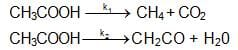What would be the % of CH4 by mole in the product mixture (excluding CH3COOH)?

Detailed Solution for P. Bahadur Test: Chemical Kinetics - Question 22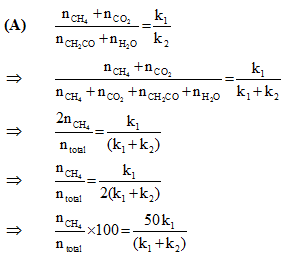P. Bahadur Test: Chemical Kinetics - Question 23

For the system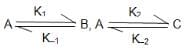which was started with only A the equilibrium concentration [A]eq is correctly related to [B]eq and [C]eq. as:

P. Bahadur Test: Chemical Kinetics - Question 24

A certain reaction A -3 B follows the given concentration (Molarity)-time graph. Which of the following statement is true?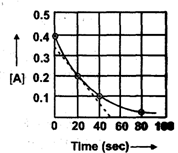Detailed Solution for P. Bahadur Test: Chemical Kinetics - Question 24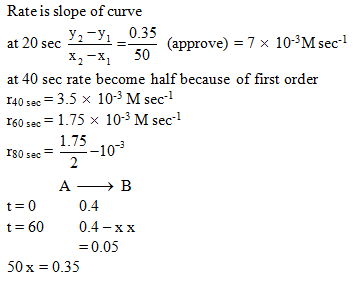P. Bahadur Test: Chemical Kinetics - Question 25

The activation energies of two reactions are Ea and Ea with Ea > EaIf temperature of the reacting systems is increased from T1 to T2, predict which of the following alternatives

Detailed Solution for P. Bahadur Test: Chemical Kinetics - Question 25

The correct  answer is option B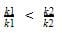Greater the activation energy of a reaction, greater is the temperature dependence of rate constant of reaction.

P. Bahadur Test: Chemical Kinetics - Question 26

A simple mechanism for enzyme-catalyzed reaction is given by the following of  equations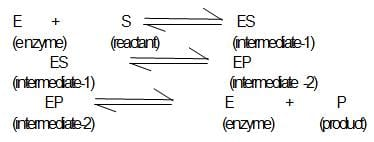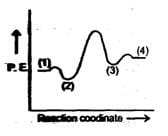This is known as the Michaleis-mechanism. The potential energy diagram is shown in the figure. Which of the following sets of identifications is correct ? (Assume that the temperature and pressure are constant).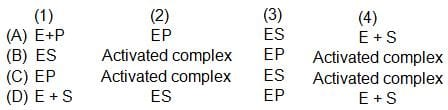Detailed Solution for P. Bahadur Test: Chemical Kinetics - Question 26

(1) E+S (2) ES (3) EP (4) E+P
Intermediate and product have less potential energy than activated complex.

P. Bahadur Test: Chemical Kinetics - Question 27

Statement-1 : If the activation energy of reaction is zero temperature will have no effect on the rate constant.

Statement-2 : Lower the activation energy fasten is the reaction.

Detailed Solution for P. Bahadur Test: Chemical Kinetics - Question 27

According to arrhenius equation K = AeEa/RT when Ea = 0, K = A.

P. Bahadur Test: Chemical Kinetics - Question 28

Statement-1 : If the order of reaction is zero than degree of dissociation will be independent upon initial concentration.

Statement-2 : The degree of dissociation of Zero order reaction is given by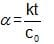Detailed Solution for P. Bahadur Test: Chemical Kinetics - Question 28

For zero order reactions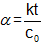They depend upon initial concentration

P. Bahadur Test: Chemical Kinetics - Question 29

Statement-1 : For a reaction A(g) → B(g)

— re = 2.5 PA           at    400K

— re = 2.5 PA           at    600K

activation energy is 4135 J/mol.

Statement-2 : Since for any reaction, values of rate constant at two different temp is same therefore activation energy of the reaction is zero.

Detailed Solution for P. Bahadur Test: Chemical Kinetics - Question 29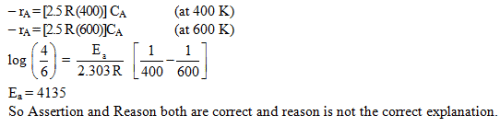P. Bahadur Test: Chemical Kinetics - Question 30

Statement-1 : For A+ 2B → C (rate = K [A]1[B]°), the half life time of reaction is only defined when conch of A and B are in stoichometric ratio

Statement-2 : For above given order half life of reaction is directly proportional to conch of A and not to conch of B due to its zero order.

Detailed Solution for P. Bahadur Test: Chemical Kinetics - Question 30

The correct answer is option C
If the concentration of B is much lower than the stoichiometric concentration, then there will not be sufficient  quantity of B to react with A and the half life period cannot be defined. Hence, the assertion is correct.
Since the reaction is first order with respect to A, the half life is independent of the concentration of A.

## Chemistry Class 12

157 videos|385 docs|261 tests
 Use Code STAYHOME200 and get INR 200 additional OFF Use Coupon Code